#### How to Summarize Survey Results in Excel

In this Excel tutorial, you will learn how to create survey and summarize survey results in Excel.

To summarize survey results in Microsoft Excel, you can use various functions and tools such as Pivot Tables, charts, and statistical functions. Here’s a basic overview of the steps to summarize survey results in Excel: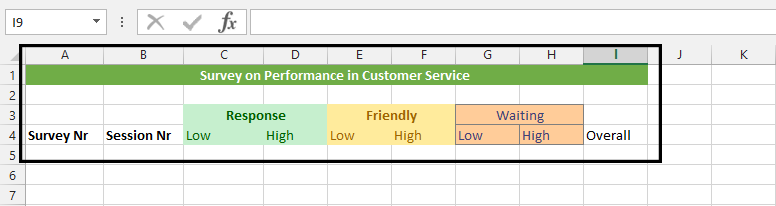Note: Response, Friendly, and Waiting are topics that have sub-topics (low and high). If someone checked 2 on a scale of 1 – 5, then that will get into low, and 3 upward will be on high. The overall is how many people participated in the survey.

## Collect the survey results in a table

2. Input the answer as they were answered by survey’s participants. Organize the data into a meaningful structure, with columns for each question and rows for each survey response.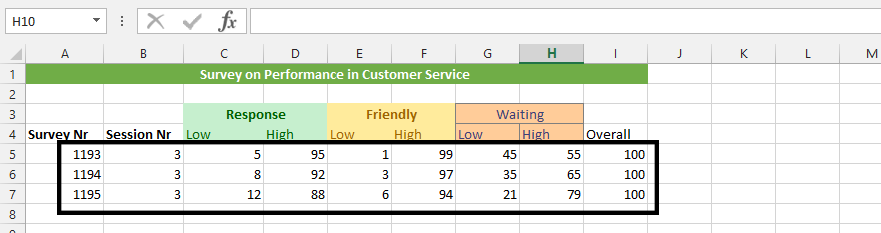3. Click on an empty cell in a, and write Total.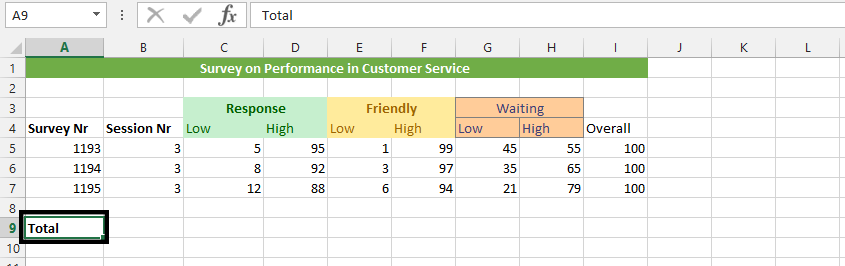4. Highlight all the cells with values, exclusive survey nr and session nr.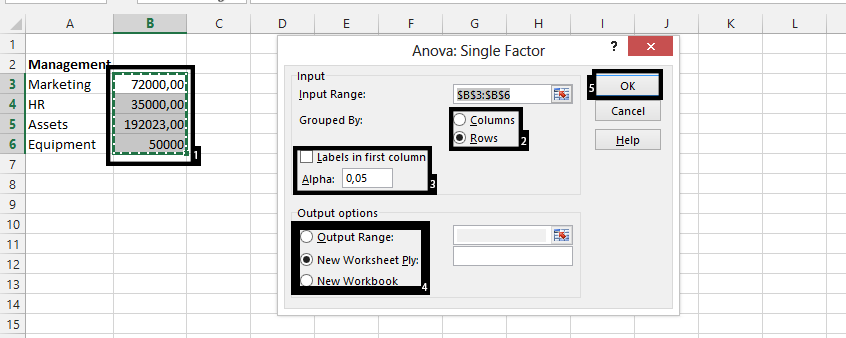## How to calculate survey results?

5. Click autosum.6. Highlight the results and press CTRL + B.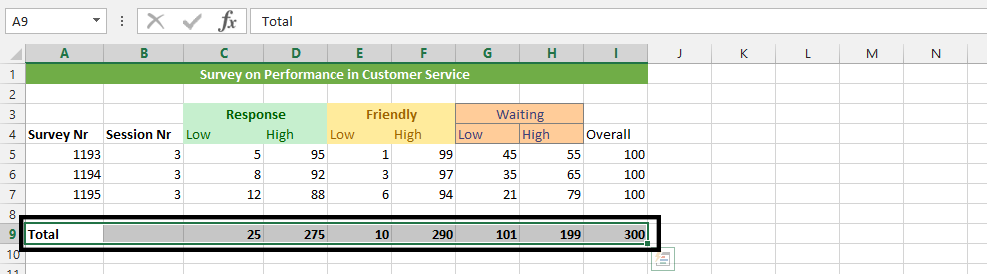We have just summarized how three hundred people sees the customer service’ performance.

Use Pivot Tables to summarize the data by grouping and aggregating the responses based on certain criteria. For example, you can create a Pivot Table to show the total number of responses for each question or to show the average rating for each question.

Use charts to visualize the survey results. For example, you can create a bar chart to show the distribution of responses for each question or a pie chart to show the percentage of responses for each option.

Use statistical functions such as AVERAGE, MEDIAN, and MODE to calculate descriptive statistics for the survey data.

Create a report or presentation to share the results of your analysis, using charts, Pivot Tables, and other visual aids to present the data in a clear and meaningful way.

Save the workbook with the summarized survey results, so that you can revisit it later or share it with others.

Note: These are just basic steps, and the exact steps may vary depending on the complexity of your survey data and the desired outcomes of your analysis.

## Key Takeaways

• To summarize survey results in Microsoft Excel, you can use various functions and tools such as Pivot Tables, charts, and statistical functions.
• You can use Pivot Tables to summarize the data by grouping and aggregating the responses based on certain criteria.
• You can use charts to visualize the survey results.
• You can use statistical functions such as AVERAGE, MEDIAN, and MODE to calculate descriptive statistics for the survey data.
• You can create a report or presentation to share the results of your analysis.

## FAQ

• Q: What are the benefits of summarizing survey results in Excel?
• A: There are many benefits to summarizing survey results in Excel. For example, you can: * Analyze the data to identify trends and patterns. * Compare the results of different surveys. * Identify areas for improvement. * Share the results with stakeholders.
• Q: What are some of the challenges of summarizing survey results in Excel?
• A: Some of the challenges of summarizing survey results in Excel include: * The data may be complex or messy. * It can be time-consuming to manually summarize the data. * It can be difficult to create charts and reports that are visually appealing and easy to understand.